0 items

# Dealing with droop

This note discusses the low-frequency behaviour of Rogowski coil current measuring systems when they are used for transient measurements. In the case of continuous currents the low-frequency limitations cause phase and amplitude errors. For transient measurements they cause a droop effect where, at the end of the transient, the the output of the measuring system does not return to zero even though the current being measured is zero.

The droop effect is mainly determined by the design of the integrator. A theoretical analysis is given for droop in both passive and active integrators. The constraints which apply in designing integrators to reduce the magnitude of the effect are described.

Finally it is shown how a measured wave-form can be 'corrected' to compensate for the droop.

### List of symbols

Symbol Description
C integrating capacitance
${C}_{3}$ blocking capacitance for AC coupling (Figure 7)
I current being measured
i integrator input current (Figure A2)
${i}_{f}$ current in the feedback resistor (Figure A2)
${i}_{c}$ current in the integrating capacitor (Figure A2)
M mutual inductance
R integrating resistance
${R}_{f}$ feedback resistance (Figure 5)
t time
$\tau$ integrator time constant (= CR)
${\mathrm{\tau }}_{1}$ low-frequency time constant for an active integrator (first time constant) = $C{R}_{f}$
${\mathrm{\tau }}_{2}$ AC coupling time constant (second time constant) =
${\mathrm{\tau }}_{3}$ combined time constant (Equation 7)
${\mathrm{V}}_{\mathrm{c}}$ coil output voltage
${\mathrm{V}}_{\mathrm{d}}$ droop voltage
${\mathrm{V}}_{\mathrm{in}}$ integrator input voltage
${\mathrm{V}}_{\mathrm{out}}$ integrator output voltage

### Introduction

A Rogowski coil measuring system consists of a coil and an integrator. In most cases it is not possible to have an integrator that works down to DC which means there will be some distortion in the measurement of the low-frequency components of the current. For continuous currents this appears as a phase shift and a reduction in amplitude at low frequencies. For transient measurements the low-frequency limitation shows as droop.

This Note is concerned with the use of analogue integrators. Although digital integrators can be used with Rogowski coils the analogue versions have considerable advantages when dealing with transient current waveforms.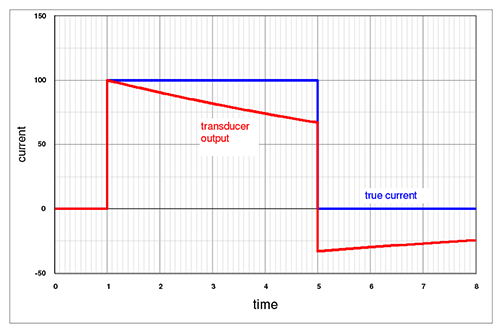Figure 1: Droop in the measurement of a rectangular pulse

Figure 1 illustrates the droop effect. It shows the response of a Rogowski coil transducer to an
idealised 'square' pulse (blue). The red curve is the transducer response. The droop rate is expressed as the percentage drop per millisecond.

At the end of the transient, when the current falls to zero the transducer output falls below the zero line. This shows that there is a droop error. In a droop-free measurement the output at the end of the transient should be zero.

For a particular integrator the droop rate depends on a time constant which is part of the design of the integrator. A short time constant causes a larger droop effect. Whether or not the droop is a problem depends on the length of the pulse compared with the integrator time constant.

### 2 Integrators

Analogue integrators can be either passive or active. Passive integrators have a short time constant but they are normally used for measuring fast pulses and in many cases the droop is not a problem. Active integrators have a much longer time constant and are able to handle low-frequency transients such as the 'DC offset' in a power-system transient.

A great advantage of Rogowski Coil systems is that the droop effect can be predicted and calculated accurately and, where necessary, corrections can be made.

The output,, of a Rogowski coil with a mutual inductance, M, depends on the rate of change of current, I:. To display the current waveform correctly this voltage must be integrated.

The output of an ideal integrator is given by:whereis the time constant of the integrator. C and R are the integrating capacitance and resistance of the integrator.

Rearranging gives:(1)

Equation 1 is the basic relationship between the voltage output of the integrator and the current being measured. It is a linear relationshipis the sensitivity of the integrator and is equivalent to the voltage across a resistor with resistance. However, with a 'real' integrator this relationship breaks down at low frequencies.

### 3 Passive Integrator

#### 3.1 Analysis

A passive integrator is the simplest system to deal with. It consists of a resistor and a capacitor as shown in Figure 2.andare the input and output voltages. It is assumed that the output is 'looking' into a high impedance.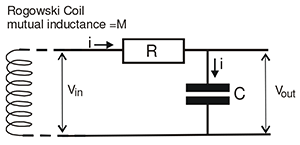Figure 2: Passive Integrator

The detailed analysis is given in Appendix 1.

The output voltage is given by:(2)

Comparing this with Equation 1 The first term on the right of equation 2 is the output of an ideal integrator with. The second term is the error due to droop.(3)

For some current-measuring devices such as current transformers, the droop is expressed as the percentage change in output per millisecond. From Appendix 1 the droop for a Rogowski coil system is related to the time constant by the relationship:(4)

#### 3.2 Design considerations

This Section looks at the possibilities for designing a passive integrator to minimise the droop effect. It can be seen from equation 3 that to minimise the droop the time constant, CR, should be as large as possible.

Rearranging Equation 1 gives:CR can be increased by using by a large value of mutual inductance, M, or by reducing the sensitivity.

#### 3.2.1 Increasing the Mutual Inductance

Unfortunately coils which have a large mutual inductance also have a high self-inductance and are not suitable for fast transients because of self-resonance effects. Each coil has a self-resonant frequency caused by the self-inductance and self-capacitance of the coil and it is not practical to make accurate measurements at frequencies near to or above the resonant frequency.

The resonant frequency depends on a number of features such as the cross-section and length of the coil former, the winding density (turns/metre) of the winding and the length of the output cable. It is not proposed to discuss these features in detail but, as a general rule, coils with a high mutual inductance also have a low resonant frequency. Flexible coils have the highest resonant frequency and can handle rise-times in the range from about 10 microseconds to less than 1 microsecond depending on the design. Rigid coils can only handle much slower rise times.

The design process involves the selection of a coil which has the highest possible mutual inductance consistent with the frequency limititations imposed by resonance effects. For 'slower' current pulses the mutual inductance can be higher, which is convenient, because these are the transients that need a low droop.

#### 3.2.2 Reducing the Sensitivity

The effect of reducing the sensitivity will be illustrated by an example. We consider a 20kA pulse with a rise-time of. A suitable coil for this measurement would have a mutual inductance of 20nH.

To get a large output signal we could choose a sensitivity of 20kA = 4V (0.2V/kA). Figure 3 shows the calculated response of the passive integrator. The blue trace is the current being measured and the red trace is the response of the coil + passive integrator. There is a considerable droop effect. The measurement error at the peak current is 6.9%.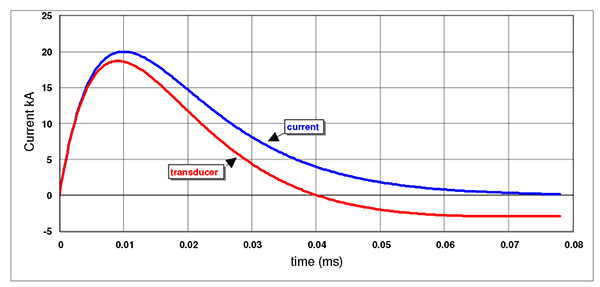Figure 3: Calculated response for a passive integrator.
sensitivity = 0.2V/kA (5kA/Volt).
time constant =The response of a passive integrator with a sensitivity of 20kA = 1V (0.05V/kA) is shown in Figure 4. In this case the droop is much less and the measurement error at peak current is only 1.8%.Figure 4: Calculated response for a passive integrator with
sensitivity = 0.05V/kA (20kA/Volt).
time constant =In choosing the sensitivity of a passive integrator system it is necessary to make a compromise between the disadvantages of a low signal level and the errors caused by droop.

### 4.1 Analysis

Figure 5 shows the basic circuit for an active integrator. A major advantage with this type of integrator is that the time constant for integration (CR) is separate from the low-frequency time constant,. By makinglarge enough the low frequency time constant can be of the order of seconds, which means they can be used at frequencies less than 1Hz, compared with milliseconds for a passive integrator.Figure 5: Active integrator

The analysis for an active integrator is given in Appendix 2.

The results are similar to a passive integrator:(5)#### 4.2 Design considerations

In the case of an active integrator the droop error is not directly related to the sensitivity except to the extent that the choice of C is influenced by the sensitivity. As with the passive integrator the aim is to makeas large as possible.

The value for C is determined mainly by the sensitivityand is usually fixed. The principal factor limiting EQUATION is the DC offset at the output of the integrator. A large value for EUATION gives a large offset. The offset can be minimised by using an integrator chip (IC1) which has a low input offset. A good practical value for the time constant is about 670ms. Figure 6 shows the response to an asymmetrical 50Hz transient for an integrator having this time constant.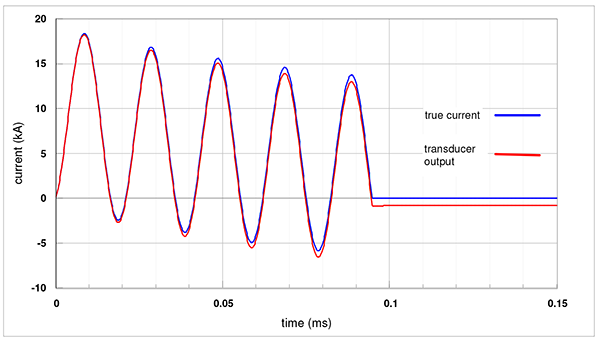Figure 6: Response to a 50Hz transient showing the droop effect with a time constant of 670ms.

#### 4.2.1 AC Coupling

The large value of needed to increase the time constant Rf and reduce the droop also causes a large DC offset at the output of the integrator. This can be eliminated by AC coupling to a buffer stage as shown in Figure 7.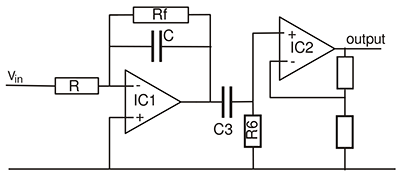Figure 7: Integrator with AC coupled second stage

This introduces a second time constant, which will influence the droop behaviour. Normally this can be made larger than the integrator time constant and its effect on the droop is less significant.

#### 4.2.2 Very Long Time Constants

Ifis made very large to increase the time constant this can degrade other aspects of integrator performance. In particular the integrator shows signs of 'low-frequency noise' where the output voltage starts to drift up and down at a low frequency with an amplitude of several millivolts or tens of millivolts. Another disadvantage of the long time constant approach is that it takes a very long time for the integrator to stabilise when it is switched on or there is a range change.

#### 4.2.3 DC Coupled Integrator

The time constant can be made 'infinite' (zero droop) by leaving out Rf altogether. In this case the integrator output will drift continuously until it saturates. It is possible to incorporate an 'offset trim' into the circuit to make the drift rate as slow as possible. A practical integrator would have a reset switch across the integrating capacitor to 'zero' the output prior to making a measurement. The reset would be released just before the transient occurs. With this arrangement it would be possible to record a short transient, such as that shown in Figure 6, with zero droop.

### 5 Droop correction

An alternative approach, which can be used in conjunction with the methods described above or instead of them, is to apply a correction to the measured wave-form. This can be used with digitally recorded waveforms from either passive or active integrators. Equation 3 gives the droop error in terms of the measured output voltage and makes it possible to apply a 'droop correction' to a recorded measurement using a simple procedure:

(i) Integrate the recorded waveform digitally and divide by the time constant.
(ii) Add the result to the original recorded waveform. The result is a droop-corrected waveform.

The time constant required for the integration can be determined from the design parameters R and C of the integrator.

For active integrators which have an AC coupled second stage (Fig 7) the correction procedure would have to be applied a second time starting with the droop-corrected waveform. Alternatively a good approximation is to use a Combined Time Constant,, and carry out the correction only once.

Ifis the time constant of the integrator stage 'first time constant' andis the time constant of the second stage 'second time constant', the combined time constant is given by:(7)

When a waveform shows droop the most obvious feature is that when the current returns to zero at the end of the transient the recorded current from the Rogowski coil and integrator (original waveform) is non-zero. If the droop correction is successful the corrected waveform should be zero at the end of the transient.

If the time constant is not known a trial-and-error approach can be used by carrying out the correction procedure for different time constants until a value is found which gives zero at the end of the transient.

### 6 Conclusions

1. A Rogowski-coil measurement system is normally not able to respond accurately to very low frequency currents. In the case of a continuous current the low-frequency limitation results in phase and amplitude errors. For transient currents it causes droop.
2. The most obvious indication of a droop problem is that when the current in a transient returns to zero the output from the integrator is non-zero.
3. The amount of droop depends on the ‘low-frequency time constant’ of the integrator. The droop is least for integrators with a long time constant.
4. For a passive integrator the low-frequency time constant is the same as the integration time constant. This type of integrator is mainly suited to high-frequency currents. For sufficiently fast transients the droop effect could be negligible.
5. For a passive integrator the low-frequency performance can be improved by using a highoutput Rogowski coil and by designing the integrator for lower sensitivity.
6. For an active integrator the low-frequency time constant is different from the integration time constant. Consequently they can be designed to work down to low frequencies less than 1Hz without placing constraints on the sensitivity.
7. The time constant of an integrator can be determined from component values used in the design of the integrator.
8. If the time constant is known the magnitude of the droop effect can be calculated reliably and accurately. For a measured waveform that shows droop it is possible to apply a correction procedure to remove the droop.

### APPENDIX 1: DROOP IN PASSIVE INTEGRATORS

A passive integrator is the simplest system to deal with. It consists of a resistor and a capacitor as shown in Figure A1.andare the input and output voltages. It is assumed that the output is 'looking' into a high impedance so that the current, i, flowing in the resistor and the capacitor are the same.,and i are all time-dependent.Figure A1: Passive Integrator

The voltage,, is given by:(A1)

The voltage across the resistor =, giving:Substituting for i into equation A1 gives:When a Rogowski coil is connected to the input,where M is the mutual inductance and I is the current being measured.

Substituting forgives:(A2)

Comparing this with Equation 1 The first term on the right of the equation is the output of an ideal integrator with. The second term is the error due to droop.

It is useful to express the droop component in a way that makes it easier to compare with other current measuring methods.

The droop voltage,, is given by:Differentiate and divide by:For a time interval,, of 1ms the fractional droop isor, whereis the integrator time constant.

### APPENDIX 2: DROOP IN ACTIVE INTEGRATORS

Referring to Figure A2 The sum of currents at the - input is zero, giving: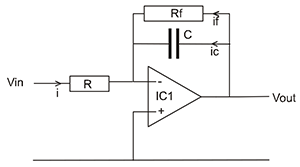Figure A2: Active Integrator

The output voltage is given by:(A3)

The - input is at zero potential: i and if are given by:Substitute these into eq. A3 to give:(A4)

The first term in eq. A4 is the ‘correct’ integration, the second term represents the droop.is the measured output. Rearrranging equation A4 gives:(A5)

The termis the amount that has to be added to correct for the droop. The productis the ’first time constant’. C andare known from the design of the integrator.

The analysis given above applies to a single-stage integrator. For an integrator with an AC-coupled second stage there is a second time constant for the AC coupling. This can be treated in a similar way.